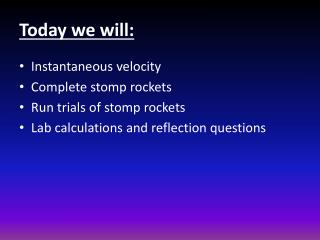DownloadDownload PresentationToday we will:

# Today we will:

Télécharger la présentation## Today we will:

- - - - - - - - - - - - - - - - - - - - - - - - - - - E N D - - - - - - - - - - - - - - - - - - - - - - - - - - -
##### Presentation Transcript

1. Today we will: • Instantaneous velocity • Complete stomp rockets • Run trials of stomp rockets • Lab calculations and reflection questions

2. Instantaneous Velocity • Instantaneous velocity is the velocity of an object at some instant or at a specific point in the object’s path.

3. Stomp Rockets • We will be measuring the maximum distance the rockets go. • We will shoot the rockets vertically and use the cross on the chapel to determine it’s vertical distance.

4. Stomp Rockets • To calculate the height of the cross (both the top of the cross and the cross bar): 1. Measure 5 m from the doors of the chapel. 2. Use the protractor to estimate the angle formed by the line parallel to the ground at your eye level and the line from the top of the object to your eyes. 3. Use your calculator to find the tangent of the angle from step two. Ex: If the angle from step two was 35 degrees, you would get approximately 0.700. 4. Multiply your distance from the object by the result from step three. Ex: You would multiply 5 m by 0.700 to get about 3.5 m. 5. Measure the distance from the ground to your eyeball and add the result to the result from step four to calculate the height of the object. Ex: If you measure 1.5 m from the ground to your eyeballs, you would add 1.5 m to 3.5m to find the total height of the object equals 5 m.

5. Stomp Rockets – Things to remember • Use the same person as the stomper for each trial. • Calculate the height (distance) of your rocket using the trig method. • Stop your stop watch at the height of the rocket’s path.